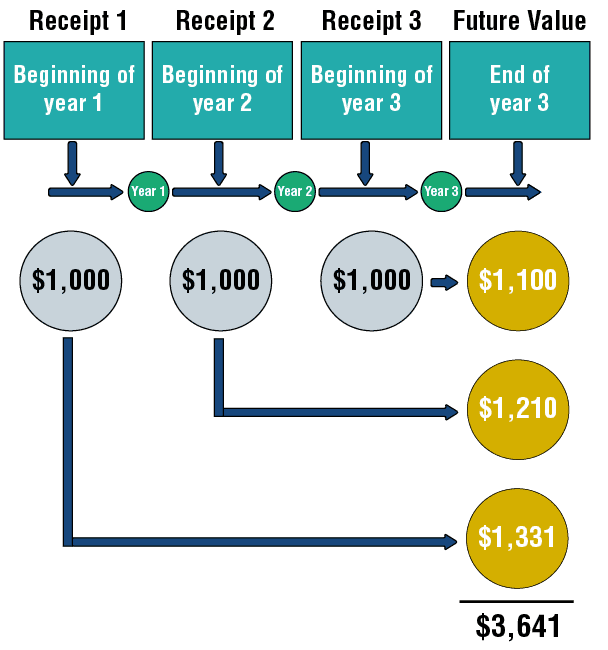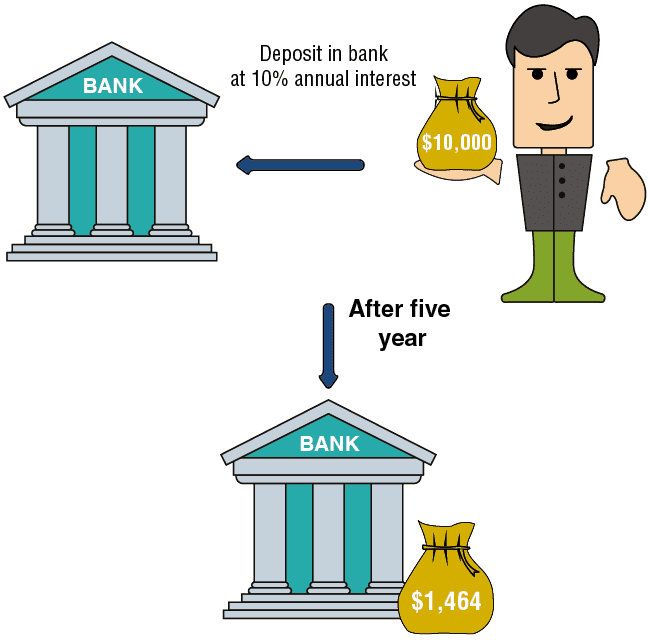# What is Future Value?

Example: A new year is beginning and a rich uncle promises to give you \$1,000 at the beginning of each year for three consecutive years. How much money will accrue to your credit at the bank at the end of three years if the entire sum is deposited in the bank at the time it is received, at 10% annual interest?

The solution method:

We will calculate the future value of each income installment. We will add the results, and we will obtain the future value of the flow of income. In this example, the future value of the flow of income will amount to \$3,641. In terms of indifference, it can be said that we are indifferent to a choice between the following two alternatives:

1. Receiving \$1,000 every year for three years.

2. Receiving \$3,641 three years from now.Examples of Future Value

Example 1:

We have recently received \$10,000, which we have deposited in a bank for five years at 10% annual interest. In five years, the sum will increase to \$16,105. \$16,105 is the future value five years from now of \$10,000 today.

Example 2:

We are due to receive \$1,000 one year from now, and this sum will be deposited in a bank for four years at 10% annual interest. In this example, \$1,464 will accrue to our credit five years from now (\$1,464 is the future value five years from now of the \$1,000 we will receive one year from now).